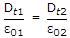# Electronics and Communication Engineering - Exam Questions Papers

6.

For dielectric to dielectric interface with surface charge density, which of the following statements are true?

1. Dn2 - Dn1 = rs
2. Et1 = Et2
3. En1 = En2
4.A. 1, 2 and 3 B. 1, 2 and 4 C. 1, 2 only D. 1, 4 only

Explanation:

No answer description available for this question. Let us discuss.

7.

Determine the correctness or otherwise of the following Assertion [a] and the Reason [r].
Assertion : FETs are more suitable at the input stages compared to BJTs
Reason: A FET has lower output impedance than a BJT.

 A. Both [a] and [r] are true and [r] is the correct reason for [a] B. Both [a] and [r] are true but [r] is not the correct reason for [a] C. Both [a] and [r] are false D. Both [a] and [r] are true

Explanation:

No answer description available for this question. Let us discuss.

8.

What are the ultimate purpose of minimizing logic expressions?

1. To get small size expression.
2. To reduce the number of variables in the given expression.
3. To implement the function of the logic expression with least hardware.
4. To reduce the expression for making it feasible for hardware implementation.
Select the correct answer from the codes given below:

 A. 1 only B. 2 and 3 C. 3 only D. 3 and 4

Explanation:

No answer description available for this question. Let us discuss.

9.

A function is given f(t) = sin2t + cos2t. Which of the following is true?

 A. f has frequency components at 0 and 1/2p Hz B. f has frequency components at 0 and 1/p Hz C. f has frequency components at 1/2p and 1/p Hz D. f has frequency components at 0, l/2p and 1/p Hz

Explanation:

No answer description available for this question. Let us discuss.

10.

The amplifier network is shown below:re and z0 is:

 A. 6.42 kΩ, 5.6 kΩ B. 6.42 Ω, (5.62) kΩ C. 6.4kΩ, (5.62) kΩ D. 6.42 Ω, 5.6 kΩ